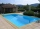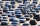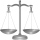# Pool

If water flows into the pool by two inlets, fill the whole for 8 hours. The first inlet filled pool 6 hour longer than second. How long pool take to fill with two inlets separately?

Result

t1 =  19.54 h
t2 =  13.54 h

#### Solution:Checkout calculation with our calculator of quadratic equations.Leave us a comment of example and its solution (i.e. if it is still somewhat unclear...):Math student
1/t1+1/(t1-10)=1/18
multiply each term by18(t1)(t1-10)
that results in
18(t1-10)+18t1=t1(t1)(t1)-10t1
using the quadratic formula results in t1=-49.6 and 3.63Dr Math
right side of equation is wrong - should be t1*(t1-10) = t12 - 10*t1 now t13-10t1Math student
the problems seems to have changed - - - t2 is now equal t1-6

therefore 1/t1+1/(t1-6)=1/18
multiplying each term by18(t1)(t1-6) ==== 18(t1-6)+18t1=t1(t1-6), simplifying further 18t1-108+18t1=t12-6t1
or 0=t12-6t1-18t1+108
graphing y=18(t1-6)+18t1-t1(t1-6) results in t1=39.25 hours and t2=39.25-6=33.25 hours (same as your NEW answer!!!!#### To solve this example are needed these knowledge from mathematics:

Looking for help with calculating harmonic mean? Looking for a statistical calculator? Looking for help with calculating roots of a quadratic equation? Do you have a linear equation or system of equations and looking for its solution? Or do you have quadratic equation? Tip: Our volume units converter will help you with converion of volume units.

## Next similar examples:

1. Three tributariesIt is possible to fill the pool with three tributaries. The first would take 12 hours, the second 15 hours, and the third 20 hours. The day before the summer season began, the manager opened all three tributaries simultaneously. How long did it take to fil55%+36%+88%+71%+100=63% what is whole (X)? Percents can be added directly together if they are taken from the same whole, which means they have the same base amount. .. . You would add the two percentages to find the total amount.
3. Intersect and conjuctionLet U={1,2,3,4,5,6} A={1,3,5} B={2,4,6} C={3,6} Find the following. 1. )AUB 2. )A'UB'
4. Car repairJohn bought a car for a certain sum of money. He spent 10% of the cost to repairs and sold the car for a profit of Rs. 11000. How much did he spend on repairs if he made a profit of 20%?
5. Angle of two linesThere is a regular quadrangular pyramid ABCDV; | AB | = 4 cm; height v = 6 cm. Determine the angles of lines AD and BV.
6. Ratio of sidesCalculate the area of a circle that has the same circumference as the circumference of the rectangle inscribed with a circle with a radius of r 9 cm so that its sides are in ratio 2 to 7.
7. Trio weightAdelka, Barunka, and Cecilka are weight in pairs. Adelka with Barunka weighs 98 kg, Barunka with Cecilka 90 kg and Adelka with Cecilka 92 kg. How much does each of them weigh?
8. Lateral surface areaThe ratio of the area of the base of the rotary cone to its lateral surface area is 3: 5. Calculate the surface and volume of the cone, if its height v = 4 cm.
9. Today in schoolThere are 9 girls and 11 boys in the class today. What is the probability that Suzan will go to the board today?
10. Rectangular trapezoidIn a rectangular trapezoid ABCD with right angles at vertices A and D with sides a = 12cm, b = 13cm, c = 7cm. Find the angles beta and gamma and height v.The radius of the sphere we reduce by 1/3 of the original radius. How much percent does the volume and surface of the sphere change?A fisherman buys carnivores to fish. He could buy either 6 larvae and 4 worms for \$ 132 or 4 larvae and 7 worms per \$ 127. What is the price of larvae and worms? Argue the answer.Find the sides of a rectangular triangle if legs a + b = 17cm and the radius of the written circle ρ = 2cm.Six workers planned to repair the roof in 5 days. After two days, two workers get sick. How long will the remaining workers complete the roof at the same rate of work?We throw two dice. What is the probability that max one three falls?Find the value of t if 2tx+5y-6=0 and 5x-4y+8=0 are perpendicular, parallel, what angle does each of the lines make with the x-axis, find the angle between the lines?The rectangular trapezoid ABCD with right angle at point B, |AC| = 12, |CD| = 8, diagonals are perpendicular to each other. Calculate the perimeter and area of ​​the trapezoid.